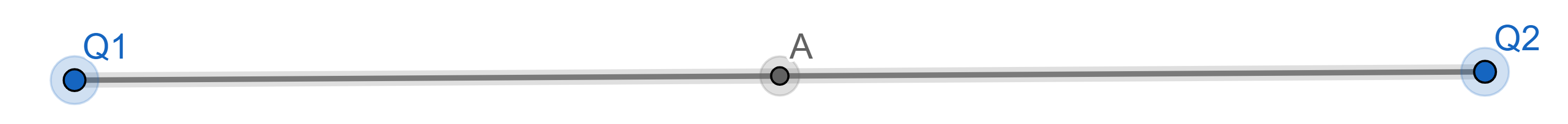EM Physics Exam 1## 第一轮复习

### 步骤

1. 写完 Practice Exam
2. 归纳错题和有疑问的题
3. 逐个解决
4. 小结

### 错题集和疑问

#### Part 1

Part 1 mainly covers:

• Coulomb’s Law
• Electric Field
• Gauss’s Law
• Electric Potential
##### Part 1 Problem 7

Question: I can deal with this problem, but why exactly?

Solution: There are two ways to solve this problem:

1. Electric potential is the integral of electric field in respect of the path. Therefore, the rate of change of electric potential over a path is electric field. Consider a function with a value of zero but a flat slope $\to$ electric potential and electric field can be both zero.

$\Delta V=-\int_a^b\vec{E}\cdot d\vec{l}$

2. Consider two identical point charges separated by a distance. Since the electric field is going to the opposite direction with the same magnitude right between the center of two charges, the net electric field goes to zero. However, by scalar addition, the point has a non-zero electric potential.##### Part 1 Problem 8

Question: same as above.

Solution: there are two way of solving this problem.

1. Same as above, but the other way around:

$\frac{dV(l)}{dl}=E(l)$

2. Consider two point charges with opposite signs and the same magnitude of charges. Since the electric field is going to the same direction with the same magnitude right between the center of two charges, the net electric field is non-zero. However, by scalar addition, the net electric potential is zero since they have the same magnitude but opposite signs.##### Part 1 Problem 9

Issue: I got it intuitively, but got the answer wrong because of the sign.

Solution: Read the question carefully and check the sign of charges carefully.

#### Part 2

Part 2 covers:

• Circuits
• Resistors
• Resistivity
• Capacitors
• Power
##### Part 2 Problem 2

Issue: I got it correctly, but I was confused by option C since I got the way of adding capacitance incorrectly.

Solution: Review.

In series: $\frac{1}{C}=\frac{1}{C_1}+\frac{1}{C_2}+\dots+\frac{1}{C_n}$

In parallel: $C=C_1+C_2+\dots+C_n$

##### Part 2 Problem 9

Issue: I accidentally put diameter as radius in calculation.

Solution: Do problems in O+P - Solve way.

## 事后

Ummm… 其实因为我懒，第二轮复习确实不知道干什么了诶，所以就直接去考试了。忘了是一周还是两周之后拿到了成绩考了个 89/100，不是很坏也不是理想的成绩！本来随随便便应该有 90+ 的，有一道题 Kiyoshi 直接没看题写了答案，因为和复习题上的有一道题几乎一样，结果教授改了一点点！然后就没有然后了…

End - of -File